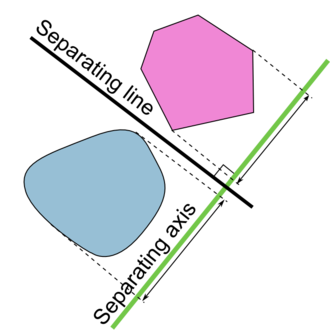# 凸多边形碰撞检测的分离轴算法（SAT）

碰撞检测可分为 Broad Phase （粗略检测）与 Narrow Phase （精细检测） 两个阶段。粗略检测阶段可直接比较两个物体的AABB包围框是否碰撞以节省计算量和时间。精细检测中，SAT（Separating Axis Theorem，分离轴定理）碰撞检测算法直观且高效，它的原理清晰易懂，即若两个物体没有发生碰撞，则总会存在一条直线，能将两个物体分离。分离轴适用的是凸多边形之间的检测，不适用于凹多边形，凹多边形的检测，可以通过算法将凹多边形分割成多个凸多边形再进行计算。算法步骤如下：

步骤一：从需要检测的多边形中取出一条边，并找出它的法向量，这个向量将会是我们的一个“投影轴”。

步骤二：循环获取第一个多边形的每个点，并将它们投影到这个轴上。

步骤三：对第二个多边形做同样的处理。

步骤四：分别得到这两个多边形的投影，并检测这两段投影是否重叠。

如果你发现了这两个投影到轴上的“阴影”有间隙，那么这两个图形一定没有相交。但如果没有间隙，那么它们则可能接触，你需要继续检测直到把两个多边形的每条边都检测完。如果你检测完每条边后，都没有发现任何间隙，那么它们是相互碰撞的。

根据上面步骤两个凸多边形之间碰撞检测的关键代码如下（参考collision.py）：

 1 def flatten_points_on(points, normal, result):
2     minpoint = math.inf
3     maxpoint = -math.inf
4
5     for i in range(len(points)):
6         dot = points[i].dot(normal)
7         if dot < minpoint:
8             minpoint = dot
9         if dot > maxpoint:
10             maxpoint = dot
11
12     result = minpoint
13     result = maxpoint
14
15
16 def is_separating_axis(a_pos, b_pos, a_points, b_points, axis):
17     range_a = [0, 0]
18     range_b = [0, 0]
19
20     offset_v = b_pos-a_pos
21
22     projected_offset = offset_v.dot(axis)
23
24     flatten_points_on(a_points, axis, range_a)
25     flatten_points_on(b_points, axis, range_b)
26
27     range_b += projected_offset
28     range_b += projected_offset
29
30     if range_a > range_b or range_b > range_a:
31         return True
32
33     return False
34
35
36 def test_aabb(b1,b2):
37     return b1 <= b2 and b2 <= b1 and b1 <= b2 and b2 <= b1
38
39
40 def test_poly_poly(a, b):
41     a_points = a.rel_points
42     b_points = b.rel_points
43     a_pos = a.pos
44     b_pos = b.pos
45
46     for n in a.normals:
47         if is_separating_axis(a_pos, b_pos, a_points, b_points, n):
48             return False
49
50     for n in b.normals:
51         if is_separating_axis(a_pos, b_pos, a_points, b_points, n):
52             return False
53
54     return True
55
56
57 def collide(a, b):
58     if not test_aabb(a.aabb, b.aabb):
59         return False
60
61     return test_poly_poly(a, b)

对于参数指定的两个凸多边形，碰撞检测函数collide先调用test_aabb来判断其AABB包围框是否相交，若外包围框不相交则证明两个多边形之间不可能发生碰撞，函数值返回False，反之则使用分离轴算法进行精细检测。函数test_poly_poly按照算法流程遍历两个多边形上的每一条边，若在某一条边上存在分离轴使得两个多边形在其上的投影不重合则证明多边形不相交，直接返回False。函数is_separating_axis根据给定的两个多边形的位置、多边形顶点的局部坐标以及分离轴向量，来计算多边形在分离轴向量上投影是否重合。

需要注意的是：对于很多图形视图和动画框架来说，图形项一般使用自己的局部坐标系来绘制，通常以其中心为原点，图形项的位置是其原点在其父图形项或者场景中的位置。分离轴判断函数中的坐标基准应一致，假如以第一个输入参数多边形$a$的位置为参考原点，则多边形$b$的顶点坐标在该参考系下，其局部坐标要加上$\overrightarrow{ab}$这个offset，因此多边形$b$在向分离轴投影时也要加上$\overrightarrow{ab}$投影后的偏移量$\overrightarrow{ab} \cdot \overrightarrow{n}$

collision.py

Hyperplane separation theorem

SAT (Separating Axis Theorem)

2D凸多边形碰撞检测算法（一） - SAT

posted @ 2021-12-04 17:25  XXX已失联  阅读(381)  评论(0编辑  收藏  举报